JMSLTM Numerical Library 7.2.0
com.imsl.math

## Class ComplexFFT

• All Implemented Interfaces:
Serializable, Cloneable

```public class ComplexFFT
extends Object
implements Serializable, Cloneable```
Complex FFT.

Class `ComplexFFT` computes the discrete complex Fourier transform of a complex vector of size N. The method used is a variant of the Cooley-Tukey algorithm, which is most efficient when N is a product of small prime factors. If N satisfies this condition, then the computational effort is proportional to N log N. This considerable savings has historically led people to refer to this algorithm as the "fast Fourier transform" or FFT.

Specifically, given an N-vector, method `forward` returns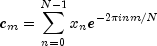Furthermore, a vector of Euclidean norm S is mapped into a vector of norm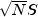Finally, note that we can invert the Fourier transform as follows: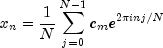This formula reveals the fact that, after properly normalizing the Fourier coefficients, one has the coefficients for a trigonometric interpolating polynomial to the data. An unnormalized inverse is implemented in `backward`. `ComplexFFT` is based on the complex FFT in FFTPACK. The package, FFTPACK was developed by Paul Swarztrauber at the National Center for Atmospheric Research.

Specifically, given an N-vector c, `backward` returns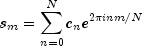Furthermore, a vector of Euclidean norm S is mapped into a vector of normFinally, note that we can invert the inverse Fourier transform as follows: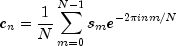This formula reveals the fact that, after properly normalizing the Fourier coefficients, one has the coefficients for a trigonometric interpolating polynomial to the data. backward is based on the complex inverse FFT in FFTPACK. The package, FFTPACK was developed by Paul Swarztrauber at the National Center for Atmospheric Research.

Example, Serialized Form
• ### Constructor Summary

Constructors
Constructor and Description
`ComplexFFT(int n)`
Constructs a complex FFT object.
• ### Method Summary

Methods
Modifier and Type Method and Description
`Complex[]` `backward(Complex[] coef)`
Compute the complex periodic sequence from its Fourier coefficients.
`Complex[]` `forward(Complex[] seq)`
Compute the Fourier coefficients of a complex periodic sequence.
• ### Methods inherited from class java.lang.Object

`clone, equals, finalize, getClass, hashCode, notify, notifyAll, toString, wait, wait, wait`
• ### Constructor Detail

• #### ComplexFFT

`public ComplexFFT(int n)`
Constructs a complex FFT object.
Parameters:
`n` - is the array size that this object can handle.
• ### Method Detail

• #### backward

`public Complex[] backward(Complex[] coef)`
Compute the complex periodic sequence from its Fourier coefficients.
Parameters:
`coef` - `Complex` array of Fourier coefficients
Returns:
`Complex` array containing the periodic sequence
• #### forward

`public Complex[] forward(Complex[] seq)`
Compute the Fourier coefficients of a complex periodic sequence.
Parameters:
`seq` - is the `Complex` array containing the sequence to be transformed.
Returns:
a `Complex` array containing the transformed sequence.
JMSLTM Numerical Library 7.2.0